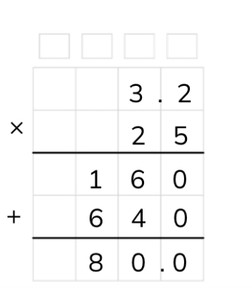Standard algorithm multiplication with one decimal number

# Standard algorithm multiplication with one decimal number

Students learn standard algorithm multiplication with one decimal number.8,000 schools use Gynzy92,000 teachers use Gynzy1,600,000 students use Gynzy

## General

The students learn standard algorithm multiplication with one decimal number.

## Standards

CCSS.Math.Content.6.NS.B.3

## Learning objective

Students will be able to multiply using the standard algorithm with decimal numbers.

## Introduction

Drag the doubles of the decimal numbers together. Example: the double of 33.48 is 66.96. In the end there are a few numbers left over that are the doubles of the doubles.

## Instruction

Explain that with standard algorithm multiplication you start by putting the numbers one under the other. You put the decimal number on top. Show that above each column there is a little box. In these boxes you can write down numbers that are regrouped to add them up with the other outcomes later on. In the intermediate step you can write down the outcomes of standard algorithm multiplication with tens and ones numbers. First imagine that the decimal point is not there and you are multiplying 2 whole numbers to each other. Explain that you begin with the lower number on the right. You multiply this number with the higher number in that column (5 × 2 = 10). From this 10 you write down the 0 under the line in the column. You can write the 1 (10) in the little box above the tens column. Now multiply the lower number from the ones column with the higher number from the tens column (5 × 3 =15). Emphasize that you shouldn't forget to add the 1 from the regrouping box to this (15 + 1 = 16). You write down this 16 under the line. This is the first outcome of the intermediate step. Next you multiply the lower number from the tens column with the higher number from the ones column (2 × 2 = 4). Then you multiply the lower number from the tens column with the higher number from the tens column (2 × 3 = 6). Now you add the numbers from the intermediate step together (160 + 640). The outcome of this is 800, but you still have to put the decimal point back. Explain that you see how many digits there are behind the decimal point in the problem, and this is how many should also be after the decimal point in the answer. Practice two problems together with the students that must be solve using the standard algorithm. The students may use scrap paper to write down their calculations. Explain that you can solve problems with multiple decimal places in the same way. Solve the problem 12.81 × 7 together with the students, step by step. The students solve the next two problems on their own.

Check whether the students can multiply using the standard algorithm with a decimal number by asking the following question:
- What steps do you take to solve the problem 15.26 × 7 using the standard algorithm?

## Quiz

The students test their understanding of standard algorithm multiplication with one decimal number through ten exercises. They must fill in the answers themselves, and can use scrap paper in order to write out their calculations.

## Closing

Discuss once again the importance of being able to multiply using the standard algorithm with one decimal number. As a closing activity the students can work in pairs to come up with problems with decimal numbers and solve them on their own. The one student names four digits and one single-digit number. The other student chooses where the decimal point should be placed in the first number. Next both students solve the problem and check their answers with one another.

## Teaching tips

Have students that have difficulty with standard algorithm multiplication with one decimal number first practice standard algorithm multiplication with numbers to 100 without a decimal number. Emphasize that the students can use the little boxes to regroup.

## Instruction materials

Scrap paper

### The online teaching platform for interactive whiteboards and displays in schools

• Save time building lessons

• Manage the classroom more efficiently

• Increase student engagement